#### 期刊菜单

Control and Prediction of Cellular Automata Epidemic Model under a Complex System
DOI: 10.12677/ORF.2017.74011, PDF, HTML, XML, 下载: 1,641  浏览: 4,174  科研立项经费支持

Abstract: Based on the complex network, we put out a CA model with medical intervention. By increasing the mobility of individual autonomy to construct dynamic social network, we simulate the transmission process of infectious disease, in the two-dimensional regular network, and depict the different time of the outbreak of the disease and flow characteristic. The propagation characteristic of different infectious diseases, such as the involuting propagation, eruption, can be reflected in the simulation. The simulation results show that: Medical intervention played a certain effect on the transmission of infectious diseases, and also gave a quantification of a medical intervention, which provided reference and basis to take reasonable prevention and intervention methods. The model can reflect the dynamic social network and individual adaptability behavior on disease transmission effect, and maintain consistency with the actual situation.

1. 引言

1) 把病情发展过程分五个阶段，建立了SEIDR传播模型，扩展了SIR模型。

2) 社会网络中研究疾病传播，借助于Philippe J.  中关于复杂系统的有关理论，在二维网络上通过增加个体的自主移动特性构建社会网络。

3) 考虑了个体的异质性和适应性行为对疾病传播的影响。

2. 模型建立

2.1. 社会网络的拓扑结构

1) 增长。初始有一个概率为 ${P}_{0}$ 的随机网络，网络的节点数比较少，在每个时间步，向网络里加入一个新节点。

2) 局部优先连接。新加入的点与已存在点的连接规则如下：首先随机连上一个点A，然后以一定的概率 ${P}_{1}$ 与点 $A$ 的邻居相连，接着以更小的概率 ${P}_{2}$ 与刚连上的 $A$ 的邻居的邻居相连。网络构建的参数设定： ${P}_{0}=0.2$${P}_{1}=0.2$${P}_{2}=0.06$ (最初为10个节点的网络，再加入5000个点)。

2.2. 元胞自动机传播模型的相关参数

2.2.1. 网络节点状态参量

1) ${S}_{k}^{t}=0$ ：易感状态 $S$ (Susceptible)，即个体未被感染，并且没有免疫力；病毒从处于易感状态 $S$ 出发，沿网络连接向四周传播。

2) ${S}_{k}^{t}=1$ ：潜伏状态 $E$ (Exposed)，病毒入侵后被感染的个体处于潜伏状态 $E$ ，由于不同个体自身对疾病的重视程度不同，处于潜伏期 $E$ 的部分个体会分别以概率 ${p}_{2},{p}_{6}$ 进入状态 $D$$I$

3) ${S}_{k}^{t}=2$ ：染病状态 $I$ (Infected)，即个体已被感染，此时个体具有传染性。

4) ${S}_{k}^{t}=3$ ：治疗状态 $D$ (Diagnosed)，患病者被确诊，并接受治疗，由于医疗作用以概率 ${p}_{5}$ 进入免疫状态，即状态5。

5) ${S}_{k}^{t}=4$ ：免疫状态 $R$ (Recovered)，在病毒传播开来后，部分处于易感状态 $S$ 的个体通过提前注射疫苗等措施对该病毒产生预免疫，进入免疫状态 $R$ 。而被感染的个体也经过一系列治疗措施，转变为免疫状态 $R$ 。个体在病毒传播期间的状态变化情况如图2所示。

2.2.2. 网络节点免疫情形

2.2.3. 网络节点传染强度

1) 潜伏期的传染强度为 ${I}_{1}$ ( $0\le {I}_{1}\le 1$ )， ${I}_{1}$ 越大，病原体越容易把疾病传染给邻元。

2) 发病期传染强度为 ${I}_{2}$ ( $0\le {I}_{2}\le 1$ )，一般情况下 ${I}_{1}<{I}_{2}$

2.3. 元胞自动机病毒传播模型的演化规则

1) 当 ${S}_{k}^{t}=0$ 时，被感染的概率 ${p}_{1}$ ，记个体在单位时间内接触的人数呈泊松分布，则个体 $k$ 单位时间内接触 $j$ 个人的概率为：

$p\left(x=j\right)=\frac{{\lambda }^{j}}{j}×{\text{e}}^{-\lambda }\left(j=0,1,2,\cdots ,{D}_{ek}\right)$

$l$ 为个体的连接矩阵，如果节点i与k相连 ${l}_{ik}=1$ 否则 ${l}_{ik}=0$ 个体被感染的概率

${p}_{i}=\underset{1\le k\le n}{\mathrm{max}}\left\{{p}_{ik}\right\}$

${p}_{ik}={l}_{ik}\underset{j=1}{\overset{{D}_{ek}}{\sum }}\frac{j}{{D}_{ek}}{I}_{x}={l}_{ik}\underset{j=1}{\overset{{D}_{ek}}{\sum }}\frac{{\lambda }^{j}}{j!}{\text{e}}^{-\lambda }\frac{j}{{D}_{ek}}{I}_{X}=\frac{{l}_{ik}{I}_{x}}{{D}_{ek}}\underset{j=1}{\overset{{D}_{ek}}{\sum }}\frac{{\lambda }^{j}{\text{e}}^{-\lambda }}{\left(j-1\right)!}$

${I}_{x}=\left\{\begin{array}{c}\begin{array}{cc}I_E& State\in E\end{array}\\ \begin{array}{cc}I_I& State\in F\end{array}\end{array}$

2) 当 ${S}_{k}^{t}=1$ 时，个体的转移概率 ${p}_{2},{p}_{6}$

${p}_{2},{p}_{6}$ 均为 $1/\left(1+{\text{e}}^{-{D}_{ek}}\right)$

3) 当 ${S}_{k}^{t}=2$ 时，计算个体状态转移概率。

4) 当 ${S}_{k}^{t}=3$ 时，计算个体的状态转移概率。

5) 当 ${S}_{k}^{t}=4$ 时，计算个体的转移概率。整个网络按照上述规则进行演化。免疫持续时间 ${g}_{t}$ 过后，免疫群体以概率 ${p}_{7}$ 返回到易感者。

3. 模拟结果与分析

3.1. 疫苗注射比率对疾病传播的影响

3.2. 接受治疗比率对疾病传播的影响

${p}_{3},{p}_{5}$ 的变化来观察疾病发展趋势，在抗病毒治疗力度( ${p}_{5}$ )确定的前提下，患病人数对染病者接受治疗的比率敏感度随着接受治疗比率提高，患病者有小幅波动下降(图6)。从爆发时间来看，接受治疗比率的增大对患病者人数增长速率的影响比较明显，接受治疗比率越大患病者增长越缓慢，最终趋于稳定，稳定值变化不明显。从仿真结果来看，单方面依赖药物治疗对传染病的控制作用仅仅是延缓了疾病的发展，并未从根本上根除疾病传播，因此应当适当治疗的同时辅助以其他控制手段。

3.3. 医疗水平对疾病传播的影响

3.4. 传染强度对疾病传播的影响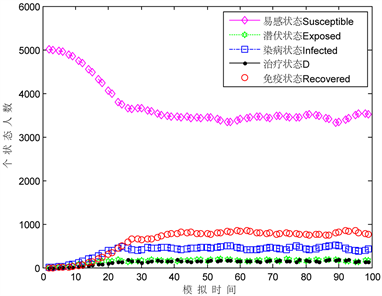Figure 1. Vaccine injection rate 0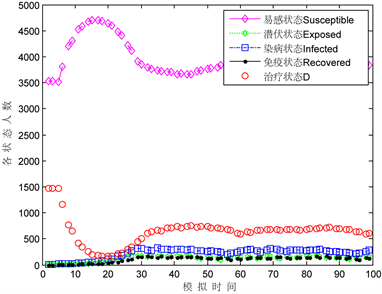Figure 2. Vaccine injection rate 0.3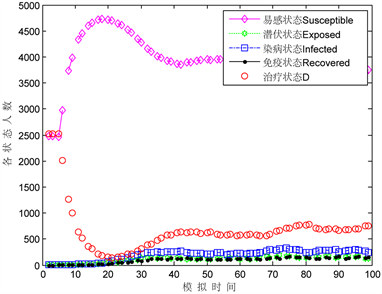Figure 3. Vaccine injection rate 0.5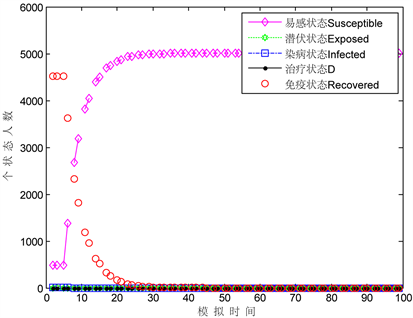Figure 4. Vaccine injection rate of 0.9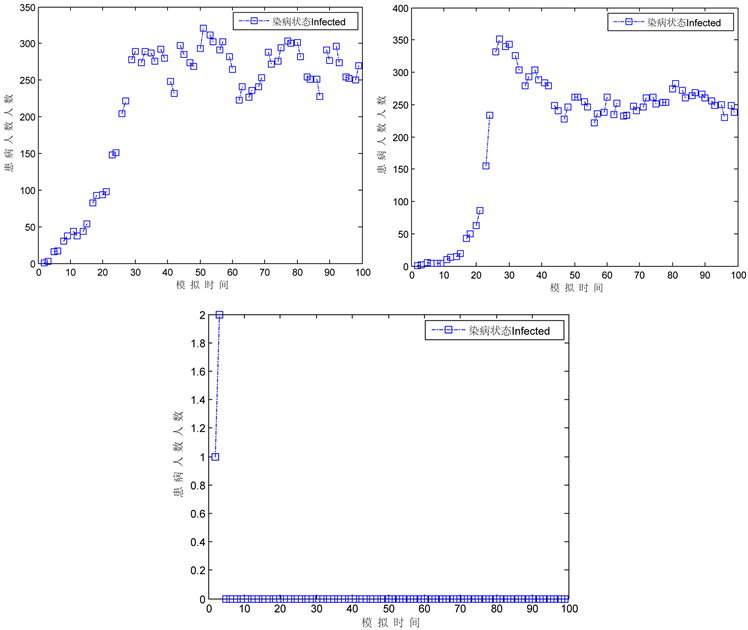Figure 5. Comparison of the patients (the injection rate was 0.1, 0.3, 0.9)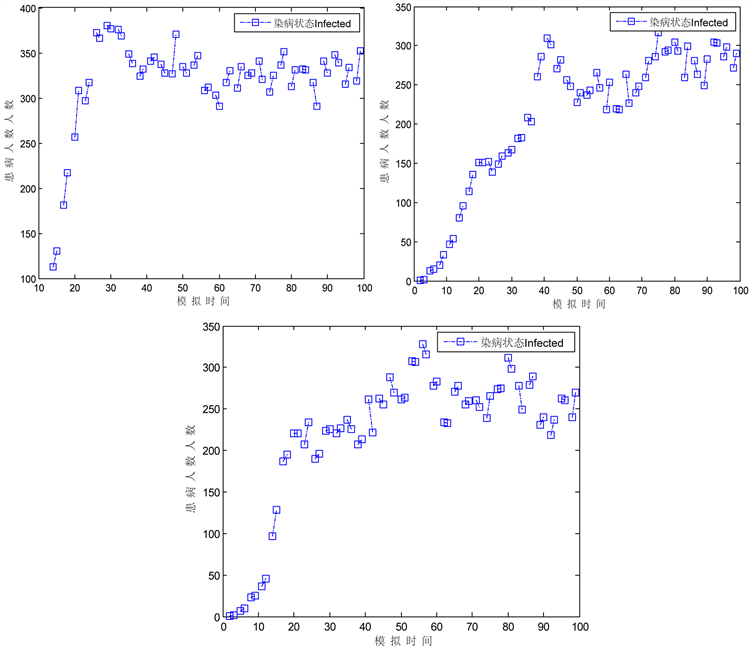Figure 6. Effects of treatment proportion on infected patients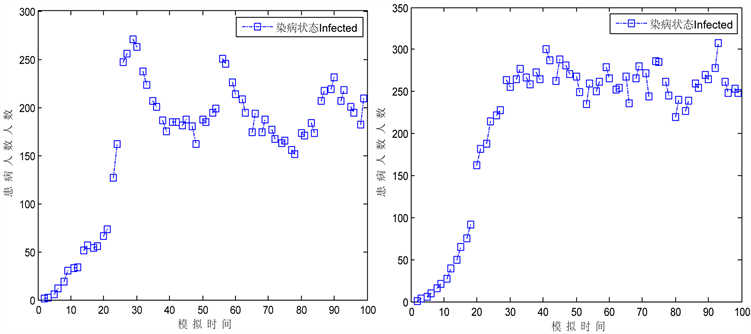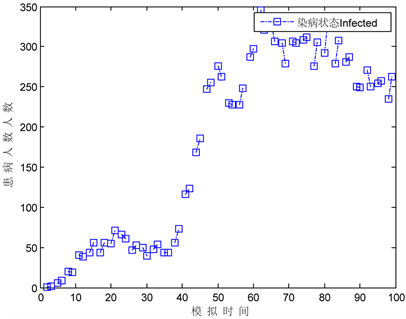Figure 7. Effect of treatment intensity on the affected population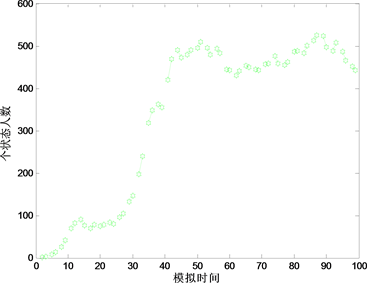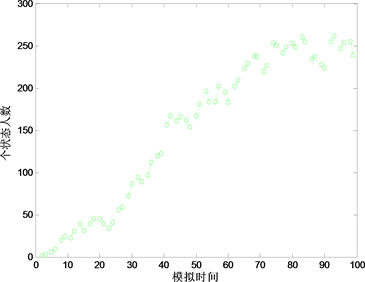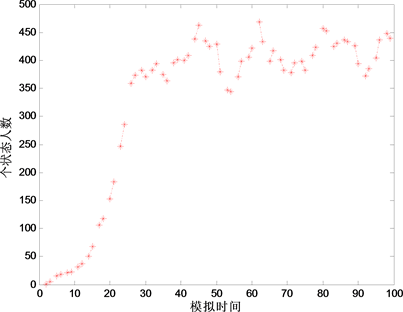Figure 8. Influence of infectious intensity on the number of people affected

3.5. 及时就医对疾病传播的影响

4. 案例分析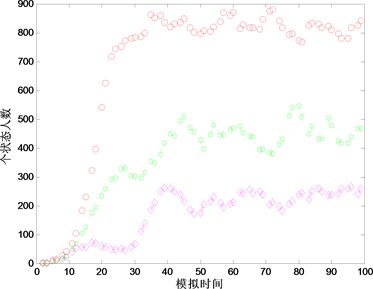Figure 9. Reaction time (1, 3, 7 days)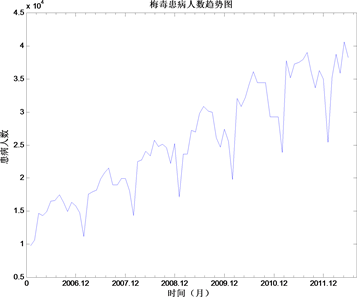Figure 10. Prevalence data of syphilis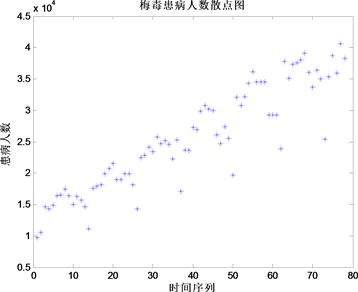Figure 11. Scatter chart of syphilis disease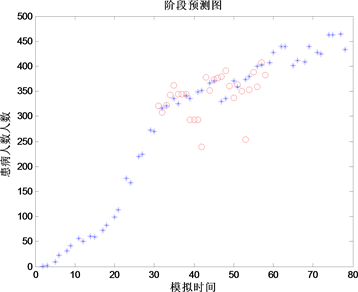Figure 12. Phase prediction chart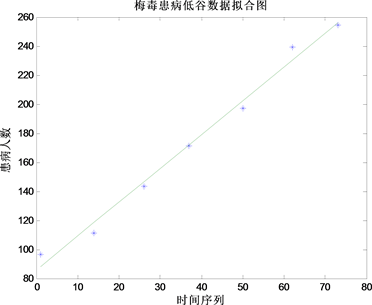Figure 13. Fit chart of the syphilis data

5. 结论

  Barker, J.L., Abrams, R.B., Ball, D.L., et al. (1983) Radiometric Calibration and Processing Procedure for Reflective Bands on Landsat-4 Protoflight Thematic Mapper. NASA Publication, Washington DC.  Desachy, J. (1985) Investigation of Landsat-4 TM Line-to-Line and Band-to-Band Registration and Telative Detector Calibration. Photogrammetric Engineering & Remote Sensing, 1291-1298.  陈宝林. 最优化理论与算法[M]. 北京: 清华大学出版社, 2002.  陈希孺. 线性模型参数的估计理论[M]. 北京: 科学出版社, 1985.  赵英时. 遥感应用分析原理和方法[M]. 北京: 科学出版社, 2003.  李璐, 宣慧玉, 高宝俊. 基于元胞自动机的异质个体HIV/AIDS传播模型[J]. 系统管理学报, 2008, 17(6): 704- 710.  关超, 彭云, 袁文燕. 基于元胞自动机带有干预机制的传染病模型[J]. 北京化工大学学报, 2011, 38(6): 109-113.  Brendan, D., Cowled, M., Garner, G., Negus, K. and Ward, M.P. (2012) Controlling Disease Outbreaks in Wildlife Using Limited Culling: Modeling Classical Swine Fever Incursions in Wild Pigs in Australia. Veterinary Research, 43, 1-3.  Peter, M.A., Sloot, A., Boukhanovsky, V. and Alfredo, W.K. (2005) A Grid-Based HIV Expert System. Journal of Clinical Monitoring and Computing, 19, 263-278. https://doi.org/10.1007/s10877-005-0673-2  Bisset, K.R., Aji, A.M. Marathe, M.V. and Feng, W.C. (2012) High-Performance Biocomputing for Simulating the Spread of Contagion over Large Contact Networks. BMC Genomics, 2012, 1471-2164. https://doi.org/10.1186/1471-2164-13-S2-S3  Chaudhury, A., Huri, P.B. and Majumder, S. (2012) Spread of Information in a Social Network Using Influential Nodes. Springer-Verlag, Berlin Heidelberg, 121-132. https://doi.org/10.1007/978-3-642-30220-6_11  Giabbanelli, P.J. (2013) A Novel Framework for Complex Networks and Chronic Diseases. Springer-Verlag, Berlin Heidelberg, 207-215. https://doi.org/10.1007/978-3-642-30287-9_22  Pastor-Satorras, R. and Vespignani, A. (2001) Epidemic Dynamical and Endemic States in Complex Networks. Physical Review E, 63, 66-117. https://doi.org/10.1103/PhysRevE.63.066117  李军, 王琳娜, 郑和义. 梅毒血清IgM抗体与传染性的关系[J]. 中国皮肤病学杂志, 2012, 7(26): 591-593.  王毅, 徐杰, 李志军, 等. 绵阳市男男性行为人群队列基线HIV/梅毒感染及影响因素分析[J]. 中国皮肤病学杂志, 2012, 5(26): 410-144.